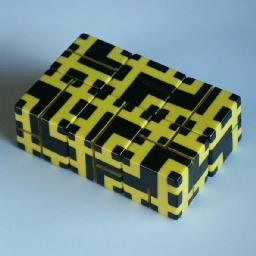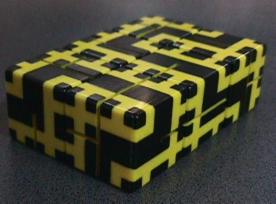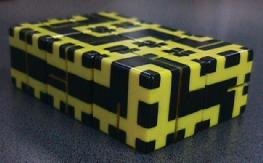# Rubik's MazeRubik's Maze consists of 6 cubes. They are connected like one long string in such a way that any face of a cube can be placed in any orientation onto any face of an adjacent cube. On the surface of the cubes is a pattern of thick black lines, separated by thin yellow ones. The aim is to place the cubes in a 2x3 rectangle in such a way that the black lines on the outside of the puzzle join up in a single long loop.

Rubik's Maze was invented by Jozsef Bognar and Ernő Rubik, 29 September 1982, DE 3,145,032.

## The number of positions:

If the cubes are numbered 1 to 6 in order, then the only restriction on the placement of the cubes in a rectangle is that cubes 1 and 2 must be adjacent, as must 2 and 3, 3 and 4 etc. This leaves only 4 distinct ways to put the 6 cubes into a 2x3 rectangle. Each cube has 24 possible orientations, giving a total of 4·246 = 764,411,904 rectangular positions of which four positions are solutions.

## Notation:

In the text below the cubes are numbered 1 to 6, where 1 is the cube which has a loop on the surface around one corner.

## Hints for finding a solution:

1. Examine the lines on each cube and examine the number of dead ends. The edges where the dead ends occur cannot become an edge of the 2x3 block.
2. The dead ends of cube 6 restrict it so much that it must be one of the central cubes in the 2x3 block, which leaves only one way of arranging the cubes, viz. cubes 3 and 6 in the middle and the others at the corners.
3. The dead ends on the surface of cube 4 determine which sides are hidden, therefore leaving it only two orientations.
4. One of the orientations of cube 4 make it impossible to place cube 5 correctly, so cube 4 is then solved.
5. Now cubes 3, 2, 1, 5 and 6 can be solved in that order, because they only have one possibility left that does not cause a dead end with the already solved cubes. Actually cubes 3 and 1 have two possibilities but the faces on the outside of the 2x3 block are the same.

## Solution:

 . . . X X X . . .
 . . . X X X . . .
 . X . X X . . . .
 . X . . X X . . .
 . . . X X . . X .
 4 . . X X . . X .
 3 . . . X X . X .
 2 . . X X . . X .
 5 X . . X . . X .
 6 X . . X . . X .
 1 X . . X X . . .
 . . . . X X . X .
 . X . X X . . . .
 . X . X X . . . .
 . X . . X X . . .
 . . . X X . . X .
 . . . X X . . X .
 . . . . X X . X .
 . X . X X . . . .
 . X . . X X . . .
 . X . X X . . . .
 . . . . X X . X .Pictures kindly supplied by Corneliu Zavadovschi.